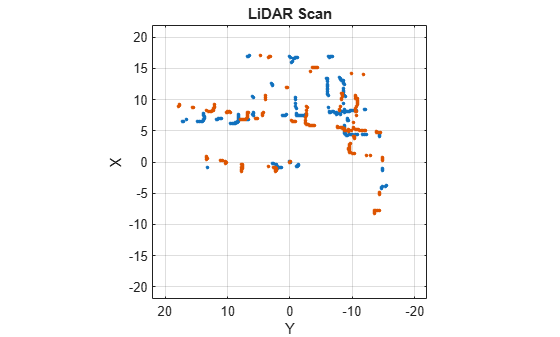# matchScansGrid

Estimate pose between two lidar scans using grid-based search

## Syntax

``pose = matchScansGrid(currScan,refScan)``
``````[pose,stats] = matchScansGrid(___)``````
``[___] = matchScansGrid(___,Name,Value)``

## Description

example

````pose = matchScansGrid(currScan,refScan)` finds the relative pose between a reference `lidarScan` and a current `lidarScan` object using a grid-based search. `matchScansGrid` converts lidar scan pairs into probabilistic grids and finds the pose between the two scans by correlating their grids. The function uses a branch-and-bound strategy to speed up computation over large discretized search windows.```
``````[pose,stats] = matchScansGrid(___)``` returns additional statistics about the scan match result using the previous input arguments.```
````[___] = matchScansGrid(___,Name,Value)` specifies options using one or more `Name,Value` pair arguments. For example, `'InitialPose',[1 1 pi/2]` specifies an initial pose estimate for scan matching.```

## Examples

collapse all

Perform scan matching using a grid-based search to estimate the pose between two laser scans. Generate a probabilistic grid from the scans and estimate the pose difference from those grids.

Load the laser scan data. These two scans are from an actual lidar sensor with changes in the robot pose and are stored as `lidarScan` objects.

```load laserScans.mat scan scan2 plot(scan) hold on plot(scan2) hold off```Use `matchScansGrid` to estimate the pose between the two scans.

`relPose = matchScansGrid(scan2,scan);`

Using the estimated pose, transform the current scan back to the reference scan. The scans overlap closely when you plot them together.

```scan2Tformed = transformScan(scan2,relPose); plot(scan) hold on plot(scan2Tformed) hold off```## Input Arguments

collapse all

Current lidar scan readings, specified as a `lidarScan` object.

Your lidar scan can contain `Inf` and `NaN` values, but the algorithm ignores them.

Reference lidar scan readings, specified as a `lidarScan` object.

Your lidar scan can contain `Inf` and `NaN` values, but the algorithm ignores them.

### Name-Value Arguments

Specify optional pairs of arguments as `Name1=Value1,...,NameN=ValueN`, where `Name` is the argument name and `Value` is the corresponding value. Name-value arguments must appear after other arguments, but the order of the pairs does not matter.

Before R2021a, use commas to separate each name and value, and enclose `Name` in quotes.

Example: `'InitialPose',[1 1 pi/2]`

Initial guess of the current pose relative to the reference laser scan, specified as the comma-separated pair consisting of `'InitialPose'` and an ```[x y theta]``` vector. `[x y]` is the translation in meters and `theta` is the rotation in radians.

Grid cells per meter, specified as the comma-separated pair consisting of `'Resolution'` and a positive integer. The accuracy of the scan matching result is accurate up to the grid cell size.

Maximum range of lidar sensor, specified as the comma-separated pair consisting of `'MaxRange'` and a positive scalar.

Search range for translation, specified as the comma-separated pair consisting of `'TranslationSearchRange'` and an `[x y]` vector. These values define the search window in meters around the initial translation estimate given in `InitialPose`. If the `InitialPose` is given as ```[x0 y0]```, then the search window coordinates are ```[x0-x x0+x]``` and `[y0-y y0+y]`. This parameter is used only when `InitialPose` is specified.

Search range for rotation, specified as the comma-separated pair consisting of `'RotationSearchRange'` and a positive scalar. This value defines the search window in radians around the initial rotation estimate given in `InitialPose`. If the `InitialPose` rotation is given as `th0`, then the search window is ```[th0-a th0+a]```, where `a` is the rotation search range. This parameter is used only when `InitialPose` is specified.

## Output Arguments

collapse all

Pose of current scan relative to the reference scan, returned as an `[x y theta]` vector, where `[x y]` is the translation in meters and `theta` is the rotation in radians.

Scan matching statistics, returned as a structure with the following field:

• `Score` — Numeric scalar representing the score while performing scan matching. This score is an estimate of the likelihood that the transformed current scan matches the reference scan. `Score` is always nonnegative. Larger scores indicate a better match, but values vary depending on the lidar data used.

• `Covariance` — Estimated covariance representing the confidence of the computed relative pose, returned as a 3-by-3 matrix.

 Hess, Wolfgang, Damon Kohler, Holger Rapp, and Daniel Andor. "Real-Time Loop Closure in 2D LIDAR SLAM." 2016 IEEE International Conference on Robotics and Automation (ICRA). 2016.